# HeatCapacitor

Lumped thermal element storing heat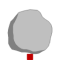# Information

This information is part of the Modelica Standard Library maintained by the Modelica Association.

This is a generic model for the heat capacity of a material. No specific geometry is assumed beyond a total volume with uniform temperature for the entire volume. Furthermore, it is assumed that the heat capacity is constant (independent of temperature).

The temperature T [Kelvin] of this component is a state. A default of T = 25 degree Celsius (= SIunits.Conversions.from_degC(25)) is used as start value for initialization. This usually means that at start of integration the temperature of this component is 25 degrees Celsius. You may, of course, define a different temperature as start value for initialization. Alternatively, it is possible to set parameter steadyStateStart to true. In this case the additional equation 'der(T) = 0' is used during initialization, i.e., the temperature T is computed in such a way that the component starts in steady state. This is useful in cases, where one would like to start simulation in a suitable operating point without being forced to integrate for a long time to arrive at this point.

Note, that parameter steadyStateStart is not available in the parameter menu of the simulation window, because its value is utilized during translation to generate quite different equations depending on its setting. Therefore, the value of this parameter can only be changed before translating the model.

This component may be used for complicated geometries where the heat capacity C is determined my measurements. If the component consists mainly of one type of material, the mass m of the component may be measured or calculated and multiplied with the specific heat capacity cp of the component material to compute C:

```   C = cp*m.
Typical values for cp at 20 degC in J/(kg.K):
aluminium   896
concrete    840
copper      383
iron        452
silver      235
steel       420 ... 500 (V2A)
wood       2500
```

# Parameters (1)

C Value: Type: HeatCapacity (J/K) Description: Heat capacity of element (= cp*m)

# Connectors (1)

 port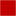Type: HeatPort_a

# Used in Examples (19)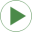HeatingMOSInverter Modelica.Electrical.Analog.Examples Heating MOS InverterHeatingNPN_OrGate Modelica.Electrical.Analog.Examples Heating NPN Or GateHeatingPNP_NORGate Modelica.Electrical.Analog.Examples Heating PNP NOR GateHeatingRectifier Modelica.Electrical.Analog.Examples Heating rectifierDCPM_Cooling Modelica.Electrical.Machines.Examples.DCMachines Test example: Cooling of a DCPM motorEddyCurrentBrake Modelica.Mechanics.Rotational.Examples Demonstrate the usage of the rotational eddy current brakeEddyCurrentBrake Modelica.Mechanics.Translational.Examples Demonstrate the usage of the translational eddy current brakeSimpleCooling Modelica.Thermal.FluidHeatFlow.Examples Simple cooling circuitParallelCooling Modelica.Thermal.FluidHeatFlow.Examples Cooling circuit with parallel branchesIndirectCooling Modelica.Thermal.FluidHeatFlow.Examples Indirect cooling circuitPumpAndValve Modelica.Thermal.FluidHeatFlow.Examples Cooling circuit with pump and valvePumpDropOut Modelica.Thermal.FluidHeatFlow.Examples Cooling circuit with drop out of pumpParallelPumpDropOut Modelica.Thermal.FluidHeatFlow.Examples Cooling circuit with parallel branches and drop out of pumpOneMass Modelica.Thermal.FluidHeatFlow.Examples Cooling of one hot massTwoMass Modelica.Thermal.FluidHeatFlow.Examples Cooling of two hot massesTwoMasses Modelica.Thermal.HeatTransfer.Examples Simple conduction demoControlledTemperature Modelica.Thermal.HeatTransfer.Examples Control temperature of a resistorMotor Modelica.Thermal.HeatTransfer.Examples Second order thermal model of a motorGenerationOfFMUs Modelica.Thermal.HeatTransfer.Examples Example to demonstrate variants to generate FMUs (Functional Mock-up Units)

# Used in Components (2)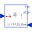DirectCapacity Modelica.Thermal.HeatTransfer.Examples.Utilities Input/output block of a direct heatCapacity model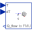InverseCapacity Modelica.Thermal.HeatTransfer.Examples.Utilities Input/output block of an inverse heatCapacity model